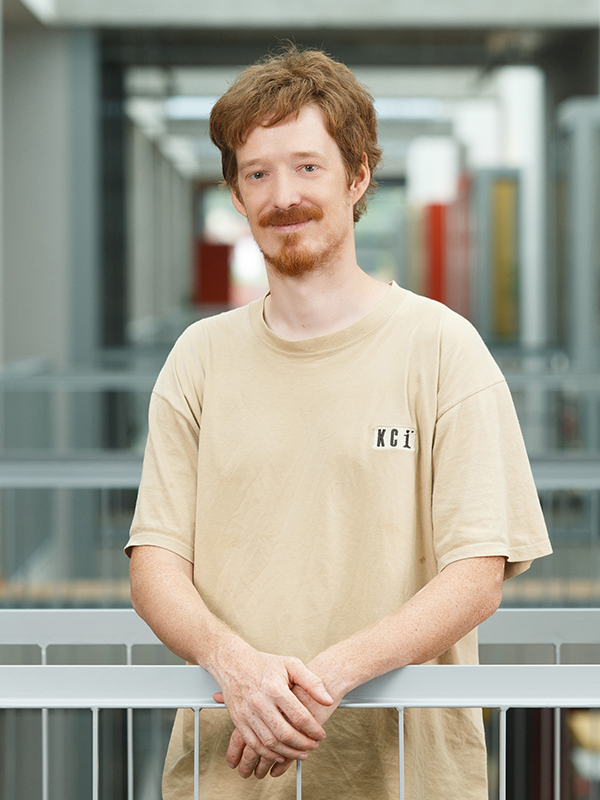• Course code:63542
• Credits:6
• Semester: winter
• Contents

Computational topology is a relatively new, but lively field somewhere between computer science and mathematics. On the mathematical side it is closely connected to topology. Topology is a somewhat loose form of geometry, where sizes, distances, angles and other numerical measures are not really important. Instead, objects are described using qualitative measures like the number of connected pieces, the number of holes of different shapes and of tunnels. Because of this, topological methods have turned out as useful in several problems where too high precision is unnecessary or even bad. Topological approaches and methods are used for example for analyzing big data sets, for modelling networks, reconstructing objects from samples, in robot motion planning, distributing tasks among processors, and so on.

The course will have a strong emphasis on student projects. Several typical problems suitable for a topological approach will be described. Through solving these problems, the basic topological concepts, structures and algorithms will be introduced, and tested on real data.

To successfully pass this course, the student has to complete 3 homework assignments, one group project, and pass a theoretical exam at the end of the semester.

• Study programmes
• Distribution of hours per semester
45
hours
lectures
20
hours
laboratory work
10
hours
tutorials
• ProfessorInstructor
Room:R3.14 - KabinetTeaching Assistant
Room:R3.26 - Laboratorij LMMRITeaching Assistant
Room:R3.26 - Laboratorij LMMRI